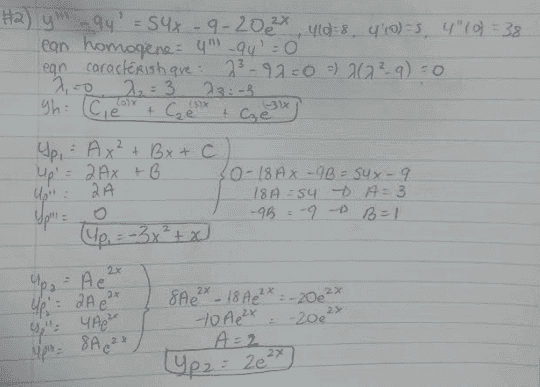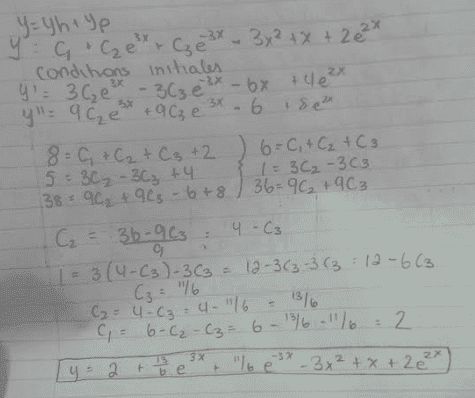# Non exact differential equation, initial value problem

• Cocoleia

## Homework Statement

I am trying to solve the following:
y'''-9y'=54x-9-20e^2x with y(0)=8, y'(0)=5, y''(0)=38

## The Attempt at a Solution